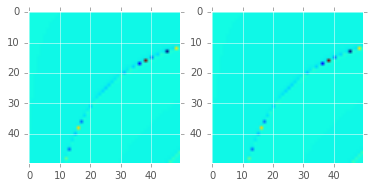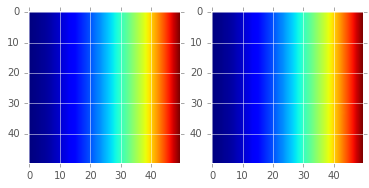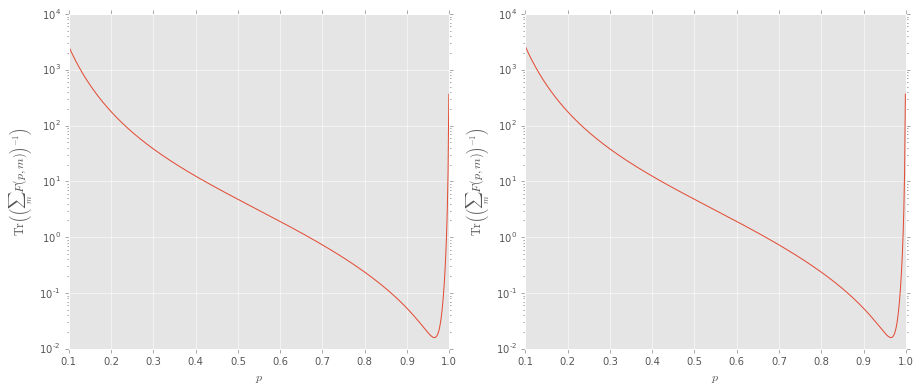# Fisher Score Mixin Example¶

This notebook demonstrates how to use the qinfer.ScoreMixin class to develop models that use numerical differentiation to calculate the Fisher information. We test the mixin class with two examples where the Fisher information is known analytically.

## Preamble¶

In :
from __future__ import division, print_function

In :
%matplotlib inline

/home/cgranade/anaconda/envs/qinfer-binder/lib/python3.5/site-packages/matplotlib/__init__.py:872: UserWarning: axes.color_cycle is deprecated and replaced with axes.prop_cycle; please use the latter.
warnings.warn(self.msg_depr % (key, alt_key))

In :
from qinfer import ScoreMixin, SimplePrecessionModel, RandomizedBenchmarkingModel
import numpy as np

/home/cgranade/anaconda/envs/qinfer-binder/lib/python3.5/site-packages/qinfer/metrics.py:51: UserWarning: Could not import scikit-learn. Some features may not work.
warnings.warn("Could not import scikit-learn. Some features may not work.")
/home/cgranade/anaconda/envs/qinfer-binder/lib/python3.5/site-packages/IPython/parallel.py:13: ShimWarning: The IPython.parallel package has been deprecated. You should import from ipyparallel instead.
"You should import from ipyparallel instead.", ShimWarning)
/home/cgranade/anaconda/envs/qinfer-binder/lib/python3.5/site-packages/qinfer/parallel.py:53: UserWarning: Could not import IPython parallel. Parallelization support will be disabled.
"Could not import IPython parallel. "

In :
import matplotlib.pyplot as plt
try:
plt.style.use('ggplot')
except:
pass


## Simple Precession Model Test¶

Create two models, one that uses ScoreMixin's numerical score method, and one that uses SimplePrecessionModel's analytic score method. To make the first model, we declare a class that does nothing but inherits from both the ScoreMixin class and SimplePrecessionModel; note that ScoreMixin is first, such that its implementation of Model.score() overrides that of SimplePrecessionModel.

In :
class NumericalSimplePrecessionModel(ScoreMixin, SimplePrecessionModel):
pass

analytic_model = SimplePrecessionModel()
numerical_model = NumericalSimplePrecessionModel()

expparams = np.linspace(1, 10, 50)
modelparams = np.linspace(.1,1,50)[:, np.newaxis]


We verify that both models compute the same score by plotting the score for a range of experiment and model parameters. Since this is a single-parameter model, the score is a scalar.

In :
analytic_score = analytic_model.score(np.array(,dtype=int),modelparams, expparams)[0,0,...]
print(analytic_score.shape)
numerical_score = numerical_model.score(np.array(,dtype=int),modelparams, expparams)[0,0,...]
print(numerical_score.shape)

plt.subplot(1,2,1)
plt.imshow(analytic_score)
plt.subplot(1,2,2)
plt.imshow(numerical_score)

(50, 50)
(50, 50)

Out:
<matplotlib.image.AxesImage at 0x7fc9bf549470>Next, we verify that both models give the same Fisher information.

In :
analytic_fisher_info = analytic_model.fisher_information(modelparams, expparams)[0,0,...]
numerical_fisher_info = numerical_model.fisher_information(modelparams, expparams)[0,0,...]

plt.subplot(1,2,1)
plt.imshow(analytic_fisher_info)
plt.subplot(1,2,2)
plt.imshow(numerical_fisher_info)

Out:
<matplotlib.image.AxesImage at 0x7fc9bf450d30>## Randomized Benchmarking Model¶

To test that we get multiparameter Fisher information calculations correct as well, we compare to the zeroth-order non-interlaced randomized benchmarking model.

In :
class NumericalRandomizedBenchmarkingModel(ScoreMixin, RandomizedBenchmarkingModel):
pass

analytic_model = RandomizedBenchmarkingModel()
numerical_model = NumericalRandomizedBenchmarkingModel()


We now make experiment and parameters to test with.

In :
expparams = np.empty((150,), dtype=analytic_model.expparams_dtype)
expparams['m'] = np.arange(1, 151)

modelparams = np.empty((500, 3))
modelparams[:, 0] = np.linspace(0.1, 0.999, 500)
modelparams[:, 1] = 0.5
modelparams[:, 2] = 0.5


Let's make sure that the returned Fisher information has the right shape. Note that the Fisher information is a four-index tensor here, with the two indices for the information matrix itself, plus two indices that vary over the input model parameters and experiment parameters.

In :
afi = analytic_model.fisher_information(modelparams, expparams)
assert afi.shape == (3, 3, modelparams.shape, expparams.shape)
nfi = numerical_model.fisher_information(modelparams, expparams)
assert nfi.shape == (3, 3, modelparams.shape, expparams.shape)


We check that each Fisher information matrix has errors that are small compared to the analytic FI alone.

In :
np.linalg.norm(afi - nfi) / np.linalg.norm(afi)

Out:
1.6626103445273564e-07

Next, we plot the trace-inverse of each to check that we get the same Cramer-Rao bounds.

In :
def tr_inv(arr):
try:
return np.trace(np.linalg.inv(arr.reshape(3, 3)))
except LinAlgError:
return float('inf')

In :
def crb(fi):
return np.apply_along_axis(tr_inv, 0, np.sum(fi.reshape((9, modelparams.shape, expparams.shape)), axis=-1))

In :
plt.figure(figsize=(15, 6))
for idx, fi in enumerate([afi, nfi]):
plt.subplot(1,2, 1 + idx)
plt.semilogy(modelparams[:, 0], crb(fi))
plt.ylabel(r'$\operatorname{Tr}\left(\left(\sum_m F(p, m)\right)^{-1}\right)$')
plt.xlabel('$p$')Finally, we note that the numerical FI calculations are not much slower than the analytic calculations.

In :
%timeit analytic_model.fisher_information(modelparams, expparams)
%timeit numerical_model.fisher_information(modelparams, expparams)

100 loops, best of 3: 18.4 ms per loop
10 loops, best of 3: 36.3 ms per loop

In [ ]: